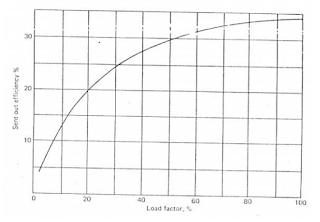# Configuring the Operating Pattern on Generating Efficiency

DUNIAPEMBANGKITLISTRIK.COM - Unit Loading. In general the overall unit efficiency will increase in line with the increase in unit loading. Figure 1, is an illustration of the correlation between unit efficiency and unit loading.Figure 1. Load Factor Graph on Efficiency

The graph in Figure 1 above, is so individual that each unit has its own graph. In the example above, it appears that the unit will have the most optimal function if it is loaded to the maximum, so that such a graph can be used as a reference when required to determine the correct configuration in the loading of operating units.

For example in a generating industry it has 3 units with a capacity of 1000 MW each. Then P2B requested that the generating industry be online on a network with a load of 2500 MW

Is one unit burdened with 1000 MW while the other 750 MW each? Or are 2 units each loaded with 2000 MW while the remaining 500 MW? So to obtain the optimum loading configuration, the graph in Figure 1 for each unit can be used as a reference.

Based on the daily load curve, demand will generally go down after midnight. For example if there are 4 units with a capacity of 100 MW each and during the daytime all operate with a total load of 350 MW. But when it was past midnight, it turned out that demand dropped to only 300 MW.

Should all four units still be operated at partial loads? Or should it only operate 3 units at full load while one unit is turned off (hot banking) to start again before dawn? If it turns out that the choice to turn off one unit is still more profitable, which unit should be turned off? The graph in Figure 1 with a comparison of startup costs can answer all these questions.

In other conditions, there are times when the power generation industry is asked to meet demand needs only for a relatively short period of time, for example, for only 2 hours. For example, for example as many as 4 units with a capacity of 100 MW each, of which 3 units operates with a maximum load to supply demand of 300 MW while 1 unit is stopped. Suddenly the demand increased by 30 MW for only ± 2 hours and had to meet those needs.

Do you have to run the unit to stop or are there other ways to meet these demands? To answer this question, data and knowledge are needed enough about the character of the unit.

Generally, each unit can be burdened with extended load. If it turns out that the extended load of the three units can meet the demand requirements, it means there is no need to run the unit that stops.

But keep in mind that extended load generally results in reduced unit efficiency. If it turns out the reduction in efficiency due to extended load for 2 hours is still more profitable than the start costs that must be
issued to run a unit that stops, then operating with extended load is an option that can be considered.

But if the extended load does not yet meet the demand, another way to think is to deactivate a final high-pressure, pre-filled pangling water heater.

In this way a higher turbine power will be obtained with
sacrifice the efficiency of the unit. But before carrying out this method must be convinced first whether boilers, turbines, generators from other assistive devices are possible to carry